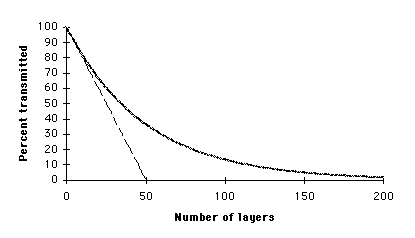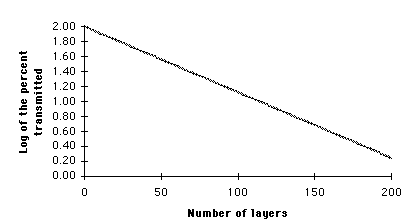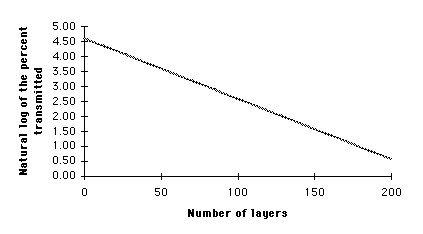##Hands On Physics

### Haze Concepts BEER'S LAW

Beer's Law is a mathematical model which describes how the intensity of light diminishes as it passes through an absorbing media. The graph below shows the percent of transmitted light as more and more layers of absorber are added in the path. This graph shows the effect of up to 200 layers of absorber.Figure B1

The figure above shows three curves, two of which are indistinguisable. The straight line represents subtracting 2% of the original light each layer. One curved line represents subtracting 2% at each layer of the light reaching that layer. The other curved line, which follows almost exactly the same curve, is a graph of the function

f(n) = 100*exp(-n*.02), where n is the number of the layer.Figure B2

This is the log to the base 10 of the percent reaching each layer, representing a graph of the log of the curved line above.Figure B3

This is the natural log (log to the base e) of the percent reaching each layer. It is also the graph of the equation g(n) = ln(f(n)) = ln(100)-.02*n = 4.605 - .02*n

Previous Page || Up a Level || Index || Next Page